# Design finite automata from regular expressions

• Last Updated : 05 Jul, 2021

Prerequisite –  Finite automata, Regular expressions, grammar, and language.

In this article, we will see some popular regular expressions and how we can convert them to finite automata (NFA and DFA). Let’s discuss it one by one.

Overview :
Let a and b are input symbols and r is the regular expression. Now we have to design NFA as well as DFA for each regular expression.

Design finite automata from the regular expression :
Here, we will discuss the Design of finite automata from regular expression as follows.

Case-1 :
When r = Φ, then FA will be as follows.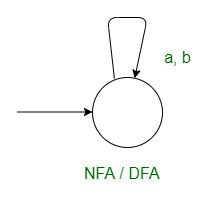Case-2 :
When r = ε, then FA will be as follows.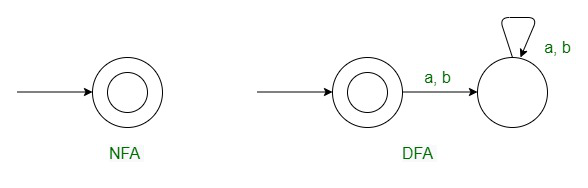Case-3 :
When r = a, then FA will be as follows.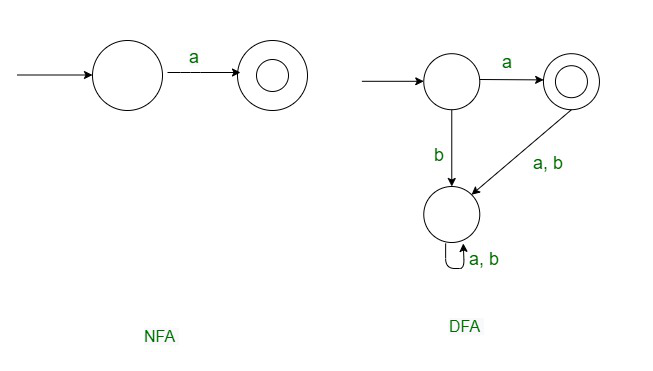Case-4 :
When r = a+b , then FA will be as follows.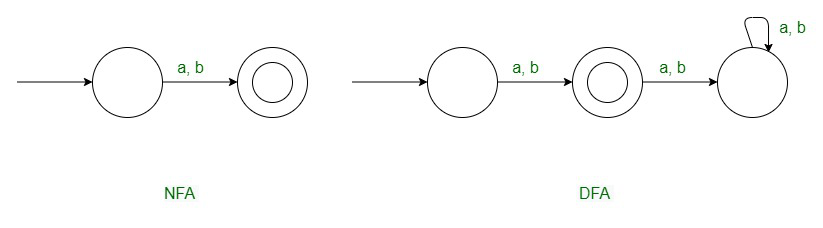Case-5 :
When r = r = a, then FA will be as follows.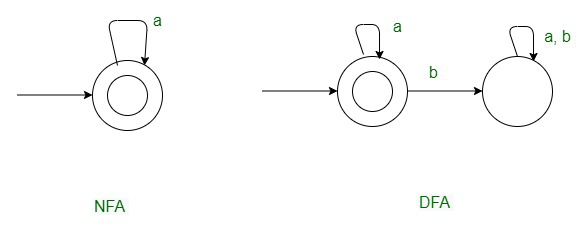Case-6 :
When r = a* + b*, then FA will be as follows.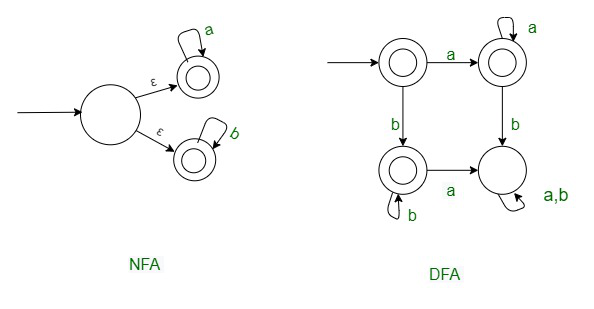Case-7 :
When r = (ab)*, then FA will be as follows.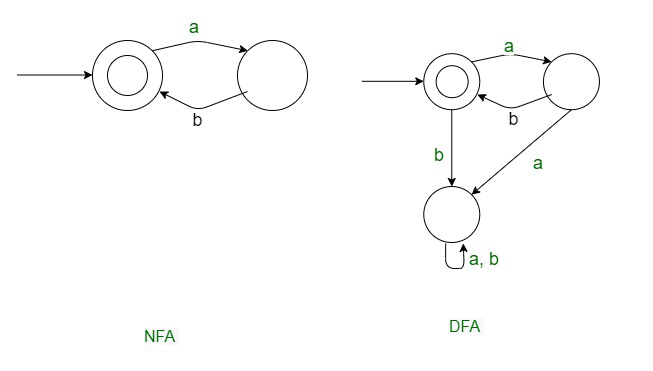Case-8 :
When r = (ab)*b, then FA will be as follows.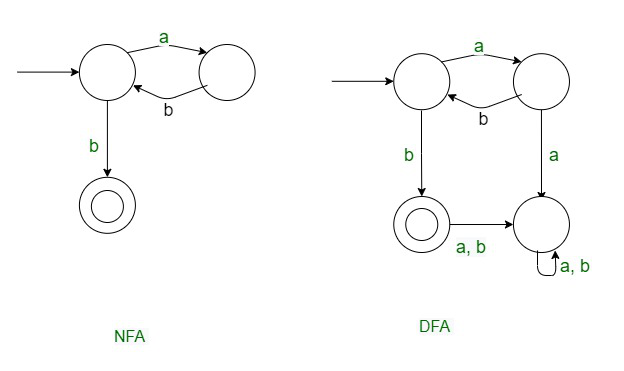Case-9 :
When r = (ab)*a, then FA will be as follows.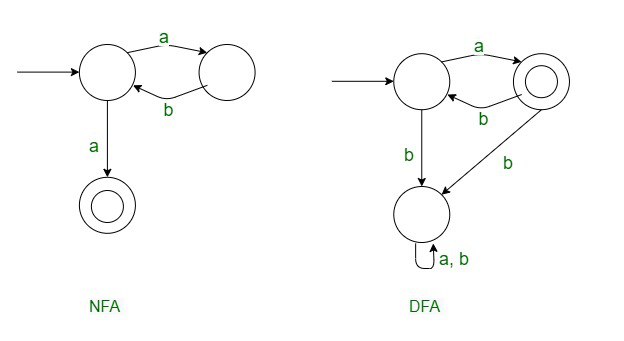Case-10 :
When r = a*b*, then FA will be as follows.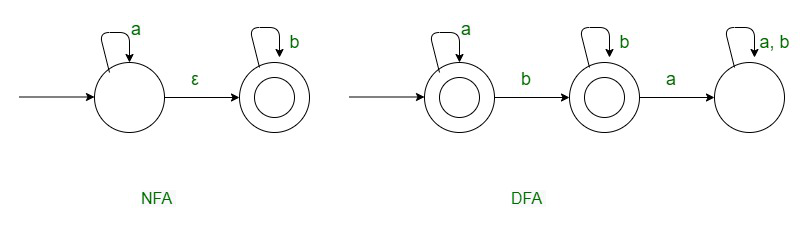Case-11 :
When r = (a+b)*, then FA will be as follows.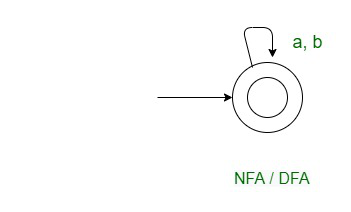Unary Design :
Let a is the input symbol and r is the regular expression. For each regular expression, we will design finite automata.

Case-1 : r = a*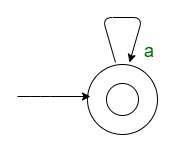Case-2 : r = (aa)*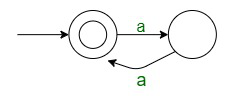Case-3 : r = (aa)*a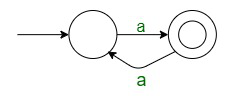Case-4 : r = aaaa*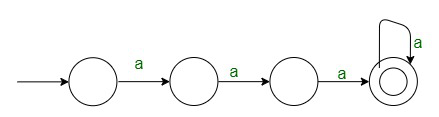Case-5 : r= (aa + aaa)*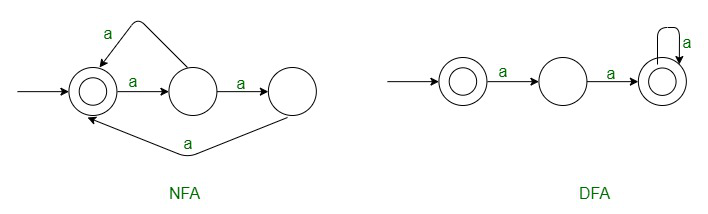Case-6 : r=( aaa+aaaaa)*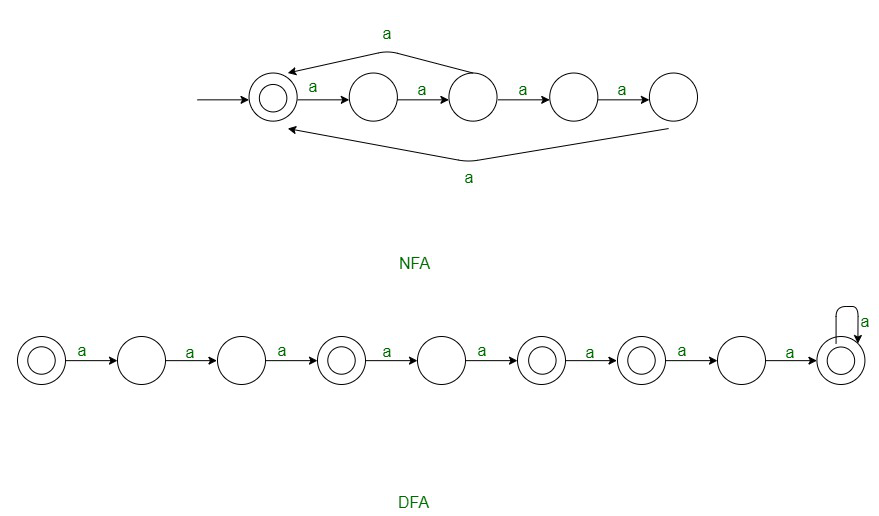Case-7 : r=(aa + aaaaaa)*
It is a multiple of 1 term i.e. aa, so it can be reduced to r=(aa)*.`L= {an | n = 7x+12, x €  Z, x >=0 }`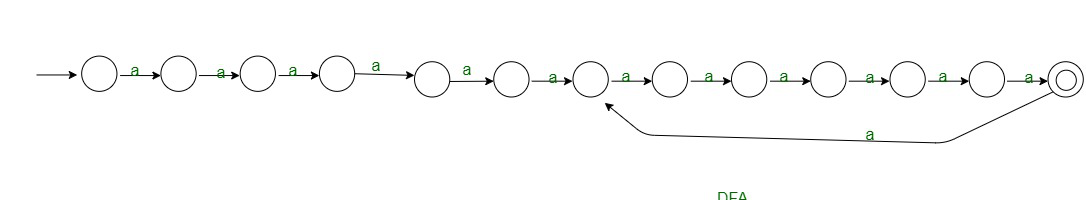General Methods :
Here, we will discuss some general methods as follows.

Method-1 :

`L = { aK1n+K2  ; n>=0 }`

If K1>K2, No. of states = K1
If K1<K2, No. of states = K2 +1
If K1=K2, No. of states = K1 +1 = k2 +1

Method-2 :

`L = { aKn  ; n>0 , K is a fixed integer }`

= { aKn  ; n>=1. K is an integer}
No. of states = K +1

Method-3 :

`L = { aKn  ; n>=0 , K is a fixed integer }`

Here, residue i.e. K2=0,  K1>K2. Therefore, No. of states =

My Personal Notes arrow_drop_up